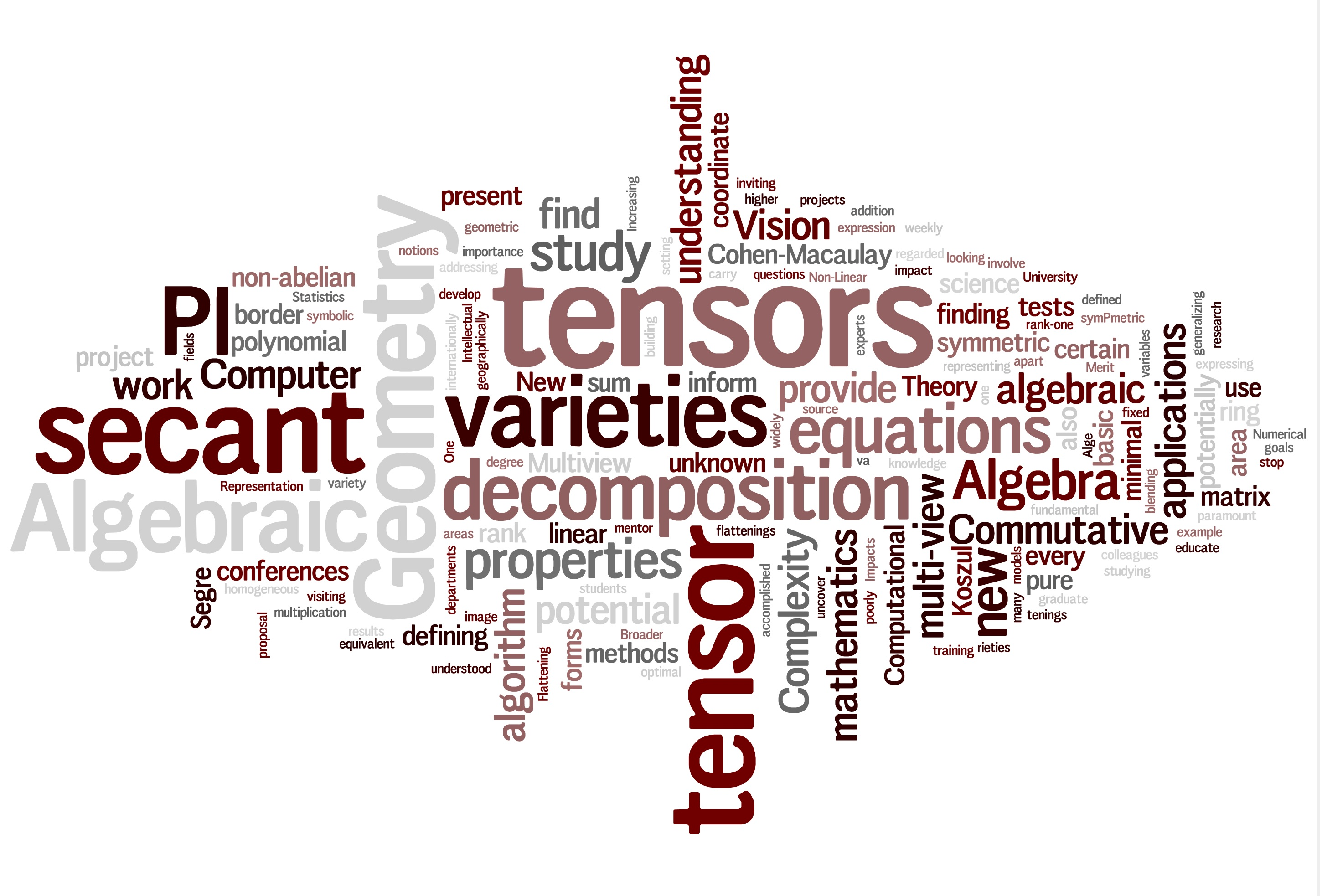# Articles by Luke Oeding

BibTeX  | Google Scholar  | AMS
Analysis of the Stochastic Alternating Least Squares Method for the Decomposition of Random Tensors, (with Yanzhao Cao, Somak Das, and Hans-Werner Van Wyk )
preprint
Learning Algebraic Models of Quantum Entanglement, (with Hamza Jaffali)
Quantum Information Processing 19, 279 (2020).
Hyperdeterminants from the $$E_8$$ discriminant, (with Frédéric Holweck)
preprint
On the (non-)vanishing of syzygies of Segre embeddings, (with Claudiu Raicu and Steven Sam)
Algebraic Geometry 6 (5) (2019) 571--591
doi:10.14231/AG-2019-026

A New Generation of Brain-Computer Interfaces Driven by Discovery of Latent EEG-fMRI Linkages using Tensor Decomposition,
Frontiers in Neuroscience, section Neural Technology 11:246.
Border Ranks of Monomials
preprint

Are all Secant Varieties of Segre Products Arithmetically Cohen-Macaulay?
preprint
Homotopy techniques for tensor decomposition and perfect identifiability (with Jon Hauenstein and Giorgio Ottaviani and Andrew Sommese)
J. Reine Angew. Math. (Crelle's Journal), Published Online Dec 2016.
Symmetrization of Principal Minors (with )
Linear and Multilinear Algebra (2016), 26 pages.
Linear Algebra and its Applications, Volume 512, 1 January 2017, Pages 306-330.
Decomposing Tensors into Frames (with Elina Robeva and Bernd Sturmfels)
Advances in Applied Mathematics 73 (2016) 125-153.
Equations for the fifth secant variety of Segre products of projective spaces (with )
Experimental Mathematics, Volume 25, Issue 1, 2016, pages 94-99.
Computations and Equations for Segre-Grassmann Hypersurfaces (with Noah Daleo and Jon Hauenstein)
Portugaliae Mathematica, Vol. 73, Fasc. 1, 2016, 71-90.
The $$3 \times 3 \times 3$$ hyperdeterminant as a polynomial in the fundamental invariants for $$SL_3(\mathbb{C}) \times SL_3(\mathbb{C}) \times SL_3(\mathbb{C})$$ (with Murray Bremner and Jiaxiong Hu)
Mathematics in Computer Science (Special Issue on Computational Algebraic Geometry), June 2014, Volume 8, Issue 2, pp 147-156.
Secant cumulants and toric geometry (with Mateusz Michalek and Piotr Zwiernik)
International Mathematics Research Notices, (2015) 12: 4019-4063.
Tangential varieties of Segre-Veronese varieties (with Claudiu Raicu)
Collectanea Mathematica, September 2014, Volume 65, Issue 3, pp 303-330.
The ideal of the trifocal variety (with Chris Aholt)
Mathematics of Computation, 83 (2014), 2553-2574.
Eigenvectors of tensors and algorithms for Waring decomposition (with Giorgio Ottaviani)
Journal of Symbolic Computation, Volume 54, July 2013, Pages 9-35.
Hyperdeterminants of polynomials
Advances in Mathematics, Volume 231, Issues 3-4, October-November 2012, Pages 1308-1326.
Secant varieties of $$\mathbb{P}^2 \times \mathbb{P}^n$$ embedded by $$\mathcal{O}(1, 2)$$ (with and Daniel Erman)
Journal of the London Mathematical Society (2012) 85 (1): 121-141.
Toward a salmon conjecture (with Dan Bates)
Experimental Mathematics, Volume 20, Issue 3, 2011, pages 358-370.
Set-theoretic defining equations of the tangential variety of the Segre variety
Journal of Pure and Applied Algebra, Volume 215, Issue 6, June 2011, Pages 1516-1527.
Set-theoretic defining equations of the variety of principal minors of symmetric matrices
Algebra and Number Theory, Vol. 5 (2011), No. 1, 75-109.
Splitting criteria for vector bundles on the symplectic isotropic Grassmannian (with )
Le Matematiche, Vol. LXIV (2009) - Fasc. II, pp. 155-176.
Dissertation: G-Varieties and principal minors of symmetric matrices (director:
Book Chapters
Weyman's method for subspace varieties of skew-symmetric tensors (with Ada Boralevi)
in J.M. Landsberg, Tensors: Geometry and Applications, (p405-407), AMS GSM, vol. 128, (2012).
Landsberg's book
Expository Work
Tensor Decomposition and Algebraic Geometry
(Simons BootCamp Lectures 2014)
Video: Lecture I, Lecture II  |  notes
Four lectures on secant varieties (with Enrico Carlini and Nathan Grieve)
Workshop on Connections Between Algebra and Geometry, Summer 2012,
PROMS (Springer Proceedings in Mathematics & Statistics), Springer/Birkhauser (2014).
AIM Report on "Geometry and representation theory of tensors for computer science, statistics and other areas."
Notes
Electric network synthesis (with Shaowei Lin and Bernd Sturmfels)
Algebraic and Geometric Aspects of Audio Signal Processing (with
Applications of Secant Varieties and their Equations (Cortona 2009)
Undergraduate Thesis: Phase space quantum mechanics (supervised by Calvin Stubbins)